M362K First Midterm Solutions, February 9, 2001

Problem 1. Continuous distributions (20 points)

A variable x is distributed continuously with probability function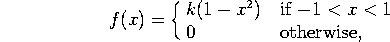where k is a normalization constant.

a) Find k.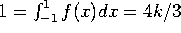, so k=3/4.

b) Find the probability that 0 < x  < 1/2.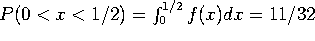Problem 2. Working Widgets (25 points)

I estimate that my brand new Wonderful Widget will last a time x, which is continuously distributed with probability function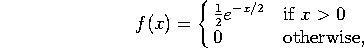where the time x is measured in years. The Wonderful Widget has a comprehensive one year warranty.

a) What is the probability the the widget will fail in the first two years?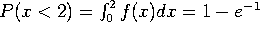.

b) If the widget fails in the first two years, what is the probability that the repairs will be covered by the warranty?

Let A be the event that it fails within 2 years, and let B be the event that it fails in the first year.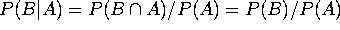. But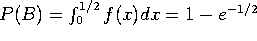, so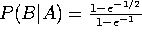.

Problem 3. Cedar Fever (25 points)

In February in Austin, there are high pollen counts about 40% of the time. If the pollen count is high, I have a 60% chance of sneezing. If the pollen count is not high, I have a 20% chance of sneezing.

a) What is the probability of my sneezing today?

Let A be the event that there is high pollen count, and let B be the event that I am sneezing.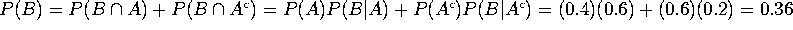.

b) If I am sneezing, what is the probability that today's pollen count is high?

P(A|B)=P(B|A)P(A)/P(B)=(0.6)(0.4)/0.36= 2/3.

Problem 4. Spinners (2 pages, 30 points)

I have a spinner that can give the number 1, 2, or 3, each with probability 1/3. I spin it twice. Let A be the event that the first result is odd. Let B be the event that the second result is odd. Let C be the event that the sum of the two spins is even.

a) Write down the sample space, and list the events A, B, and C. Your answer should be of the form ``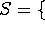(list of elements)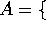(shorter list of elements)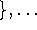''.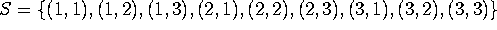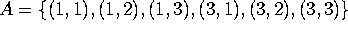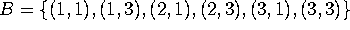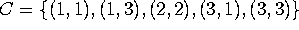b) Find P(A), P(B), P(C),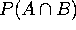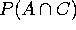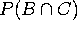, and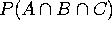. You can assume that the two spins are independent of one another.

Since the two spins are independent, every point in the sample space has probability 1/9. Events A and B each have 6 elements, while C has 5 elements.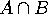has 4 elements, as do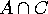and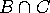. In fact,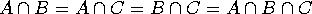. So

P(A)=P(B)=2/3, P(C)=5/9,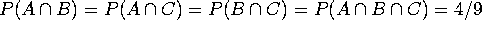.

c) Are A and B independent? Are A and C independent? Are B and C independent? Explain.

Since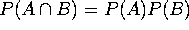, A and B are independent. Since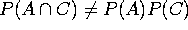, A and C are not independent. Since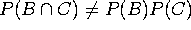, B and C are not independent.

d) Are A, B, and C independent? Explain.

No, since A and C are not independent. (You could also say no because B and C are not independent, or because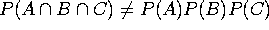.)